Ex 2.1

Chapter 2 Class 7 Fractions and Decimals
Serial order wise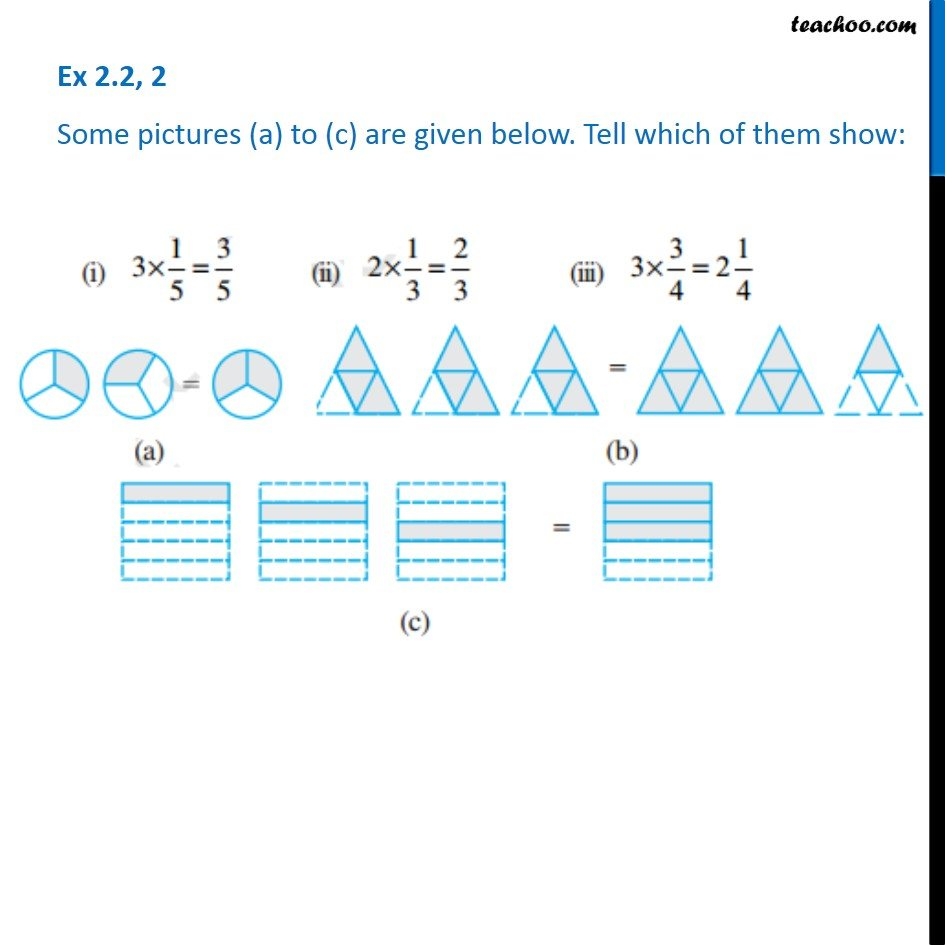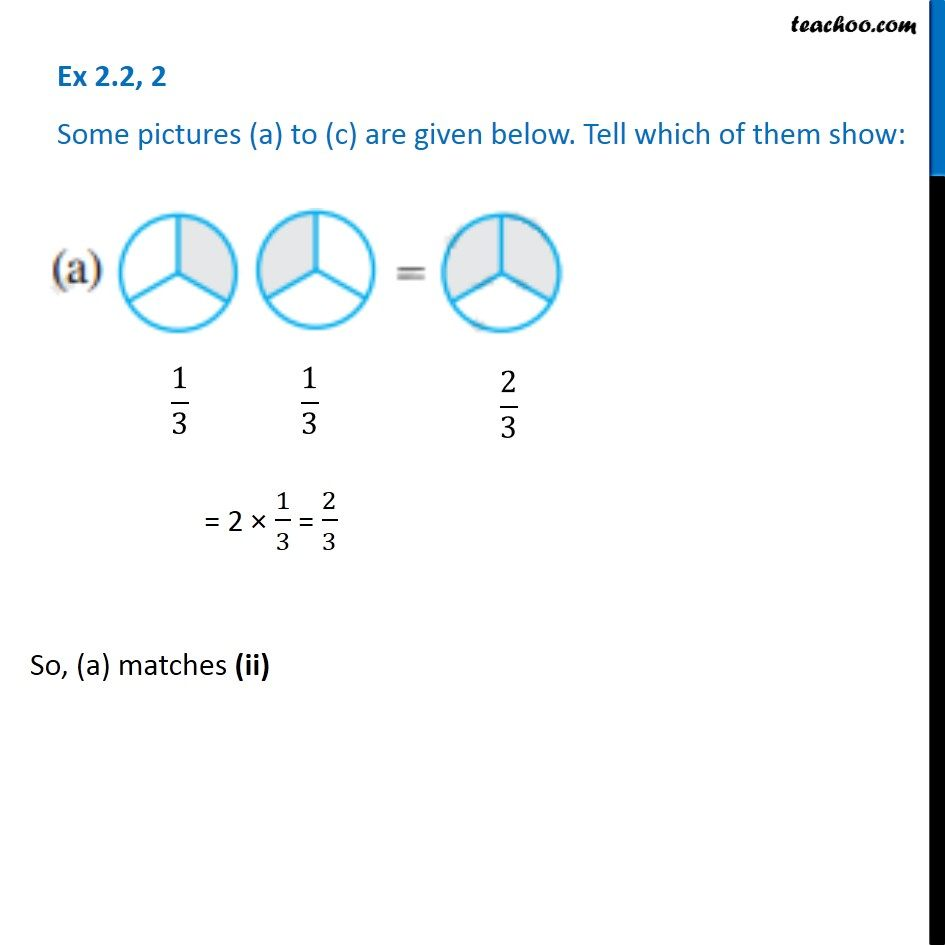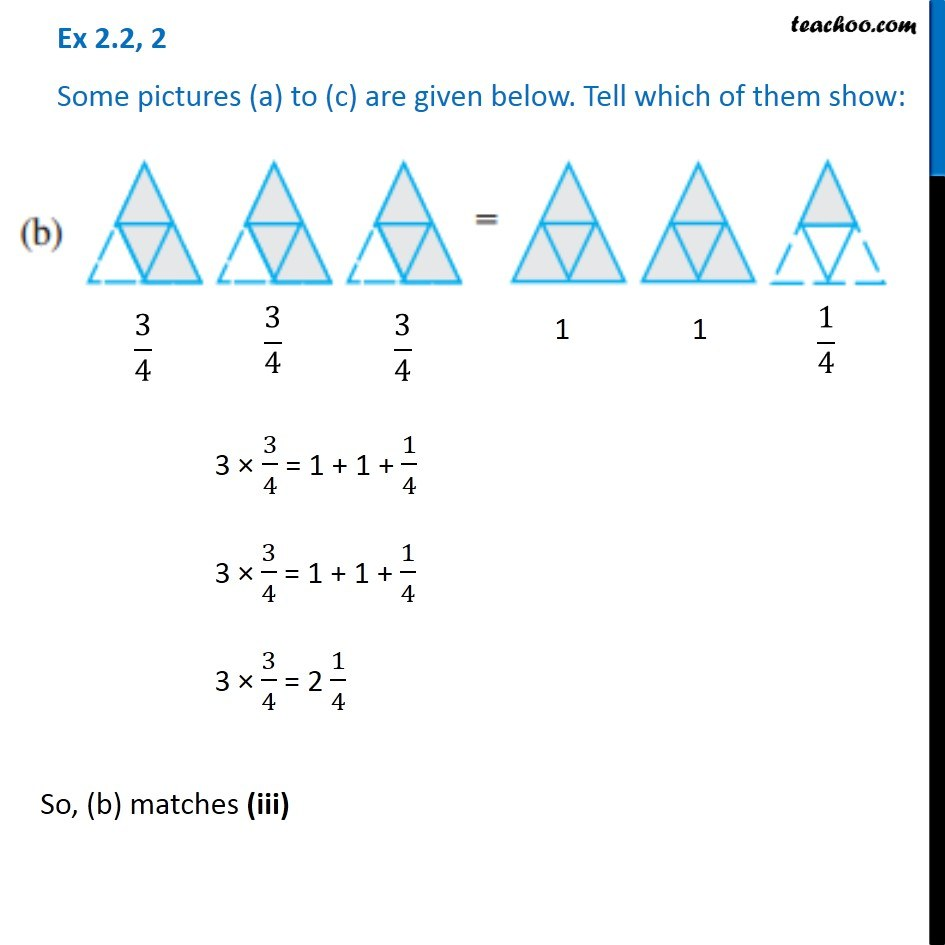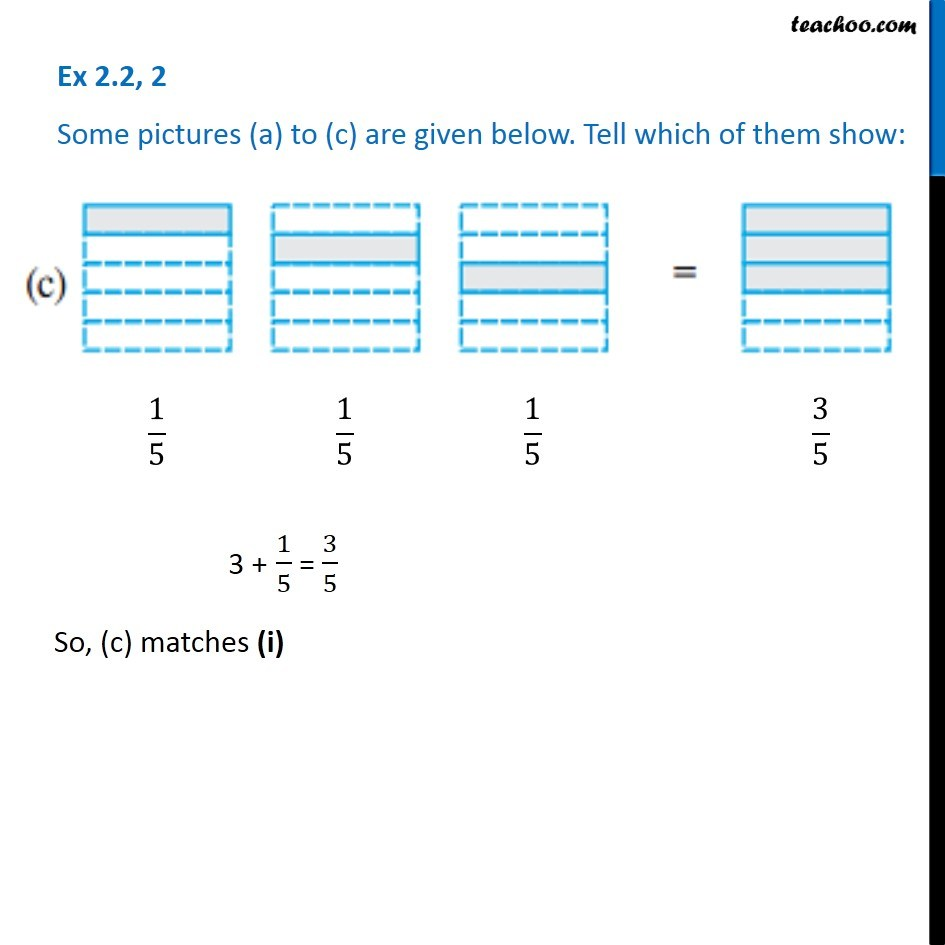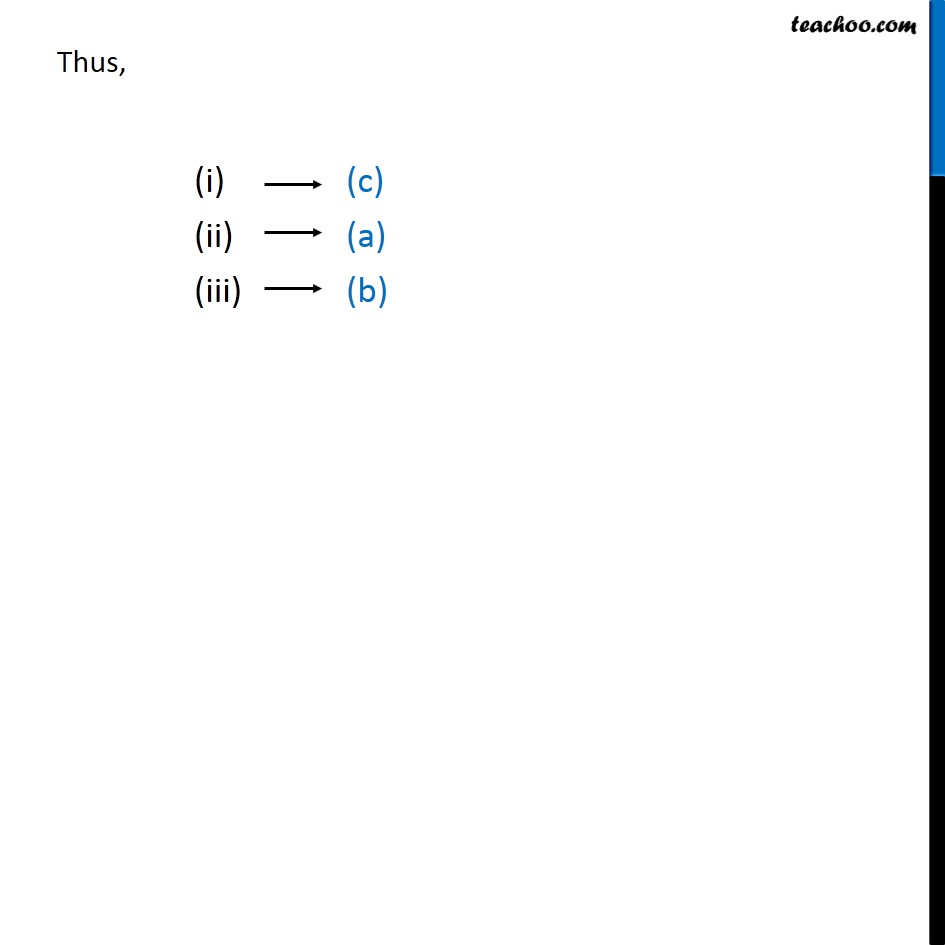Learn in your speed, with individual attention - Teachoo Maths 1-on-1 Class

### Transcript

Ex 2.1, 2 Some pictures (a) to (c) are given below. Tell which of them show: Ex 2.1, 2 Some pictures (a) to (c) are given below. Tell which of them show: So, (a) matches (ii) Ex 2.1, 2 Some pictures (a) to (c) are given below. Tell which of them show: 3 × 3/4 = 1 + 1 + 1/4 3 × 3/4 = 1 + 1 + 1/4 3 × 3/4 = 2 1/4 So, (b) matches (iii) Ex 2.1, 2 Some pictures (a) to (c) are given below. Tell which of them show:3 + 1/5 = 3/5 So, (c) matches (i) Thus,